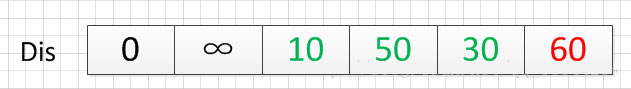# 詳解Dijkstra算法之最短路徑問題

## 一、最短路徑問題介紹

• 迪傑斯特拉算法（Dijkstra算法）
• 弗洛伊德算法（Floyd算法）
• SPFA算法

## 二、Dijkstra算法介紹

### 2.2、算法的思路

Dijkstra算法采用的是一種貪心的策略，聲明一個數組dis來保存源點到各個頂點的最短距離和一個保存已經找到瞭最短路徑的頂點的集合：T，初始時，原點 s 的路徑權重被賦為 0 （dis[s] = 0）。若對於頂點 s 存在能直接到達的邊（s,m），則把dis[m]設為w（s, m）,同時把所有其他（s不能直接到達的）頂點的路徑長度設為無窮大。初始時，集合T隻有頂點s。

## 三、Dijkstra算法示例演示OK，既然確定瞭一個頂點的最短路徑，下面我們就要根據這個新入的頂點V3會有出度，發現以v3 為弧尾的有： < v3,v4 >,那麼我們看看路徑：v1–v3–v4的長度是否比v1–v4短，其實這個已經是很明顯的瞭，因為dis代表的就是v1–v4的長度為無窮大，而v1–v3–v4的長度為：10+50=60，所以更新dis的值,得到如下結果：v1    v2     無          ∞

v3     {v1,v3}    10

v4     {v1,v5,v4}  50

v5     {v1,v5}    30

v6     {v1，v5,v4,v6} 60

## 四、Dijkstra算法的代碼實現（c++）

Dijkstra.h文件的代碼

```//@盡量寫出完美的程序

#pragma once
//#pragma once是一個比較常用的C/C++雜註，
//隻要在頭文件的最開始加入這條雜註，
//就能夠保證頭文件隻被編譯一次。

#include<iostream>
#include<string>
using namespace std;

/*

*/
//記錄起點到每個頂點的最短路徑的信息
struct Dis {
string path;
int value;
bool visit;
Dis() {
visit = false;
value = 0;
path = "";
}
};

class Graph_DG {
private:
int vexnum;   //圖的頂點個數
int edge;     //圖的邊數
int **arc;   //鄰接矩陣
Dis * dis;   //記錄各個頂點最短路徑的信息
public:
//構造函數
Graph_DG(int vexnum, int edge);
//析構函數
~Graph_DG();
// 判斷我們每次輸入的的邊的信息是否合法
//頂點從1開始編號
bool check_edge_value(int start, int end, int weight);
//創建圖
void createGraph();
//打印鄰接矩陣
void print();
//求最短路徑
void Dijkstra(int begin);
//打印最短路徑
void print_path(int);
};```

Dijkstra.cpp文件的代碼

```#include"Dijkstra.h"

//構造函數
Graph_DG::Graph_DG(int vexnum, int edge) {
//初始化頂點數和邊數
this->vexnum = vexnum;
this->edge = edge;
//為鄰接矩陣開辟空間和賦初值
arc = new int*[this->vexnum];
dis = new Dis[this->vexnum];
for (int i = 0; i < this->vexnum; i++) {
arc[i] = new int[this->vexnum];
for (int k = 0; k < this->vexnum; k++) {
//鄰接矩陣初始化為無窮大
arc[i][k] = INT_MAX;
}
}
}
//析構函數
Graph_DG::~Graph_DG() {
delete[] dis;
for (int i = 0; i < this->vexnum; i++) {
delete this->arc[i];
}
delete arc;
}

// 判斷我們每次輸入的的邊的信息是否合法
//頂點從1開始編號
bool Graph_DG::check_edge_value(int start, int end, int weight) {
if (start<1 || end<1 || start>vexnum || end>vexnum || weight < 0) {
return false;
}
return true;
}

void Graph_DG::createGraph() {
cout << "請輸入每條邊的起點和終點（頂點編號從1開始）以及其權重" << endl;
int start;
int end;
int weight;
int count = 0;
while (count != this->edge) {
cin >> start >> end >> weight;
//首先判斷邊的信息是否合法
while (!this->check_edge_value(start, end, weight)) {
cout << "輸入的邊的信息不合法，請重新輸入" << endl;
cin >> start >> end >> weight;
}
//對鄰接矩陣對應上的點賦值
arc[start - 1][end - 1] = weight;
//無向圖添加上這行代碼
//arc[end - 1][start - 1] = weight;
++count;
}
}

void Graph_DG::print() {
cout << "圖的鄰接矩陣為：" << endl;
int count_row = 0; //打印行的標簽
int count_col = 0; //打印列的標簽
//開始打印
while (count_row != this->vexnum) {
count_col = 0;
while (count_col != this->vexnum) {
if (arc[count_row][count_col] == INT_MAX)
cout << "∞" << " ";
else
cout << arc[count_row][count_col] << " ";
++count_col;
}
cout << endl;
++count_row;
}
}
void Graph_DG::Dijkstra(int begin){
//首先初始化我們的dis數組
int i;
for (i = 0; i < this->vexnum; i++) {
//設置當前的路徑
dis[i].path = "v" + to_string(begin) + "-->v" + to_string(i + 1);
dis[i].value = arc[begin - 1][i];
}
//設置起點的到起點的路徑為0
dis[begin - 1].value = 0;
dis[begin - 1].visit = true;

int count = 1;
//計算剩餘的頂點的最短路徑（剩餘this->vexnum-1個頂點）
while (count != this->vexnum) {
//temp用於保存當前dis數組中最小的那個下標
//min記錄的當前的最小值
int temp=0;
int min = INT_MAX;
for (i = 0; i < this->vexnum; i++) {
if (!dis[i].visit && dis[i].value<min) {
min = dis[i].value;
temp = i;
}
}
//cout << temp + 1 << "  "<<min << endl;
//把temp對應的頂點加入到已經找到的最短路徑的集合中
dis[temp].visit = true;
++count;
for (i = 0; i < this->vexnum; i++) {
//註意這裡的條件arc[temp][i]!=INT_MAX必須加，不然會出現溢出，從而造成程序異常
if (!dis[i].visit && arc[temp][i]!=INT_MAX && (dis[temp].value + arc[temp][i]) < dis[i].value) {
//如果新得到的邊可以影響其他為訪問的頂點，那就就更新它的最短路徑和長度
dis[i].value = dis[temp].value + arc[temp][i];
dis[i].path = dis[temp].path + "-->v" + to_string(i + 1);
}
}
}

}
void Graph_DG::print_path(int begin) {
string str;
str = "v" + to_string(begin);
cout << "以"<<str<<"為起點的圖的最短路徑為：" << endl;
for (int i = 0; i != this->vexnum; i++) {
if(dis[i].value!=INT_MAX)
cout << dis[i].path << "=" << dis[i].value << endl;
else {
cout << dis[i].path << "是無最短路徑的" << endl;
}
}
}```

main.cpp文件的代碼

```#include"Dijkstra.h"

//檢驗輸入邊數和頂點數的值是否有效，可以自己推算為啥：
//頂點數和邊數的關系是：((Vexnum*(Vexnum - 1)) / 2) < edge
bool check(int Vexnum, int edge) {
if (Vexnum <= 0 || edge <= 0 || ((Vexnum*(Vexnum - 1)) / 2) < edge)
return false;
return true;
}
int main() {
int vexnum; int edge;

cout << "輸入圖的頂點個數和邊的條數：" << endl;
cin >> vexnum >> edge;
while (!check(vexnum, edge)) {
cout << "輸入的數值不合法，請重新輸入" << endl;
cin >> vexnum >> edge;
}
Graph_DG graph(vexnum, edge);
graph.createGraph();
graph.print();
graph.Dijkstra(1);
graph.print_path(1);
system("pause");
return 0;
}```

6 8

1 3 10

1 5 30

1 6 100

2 3 5

3 4 50

4 6 10

5 6 60

5 4 20